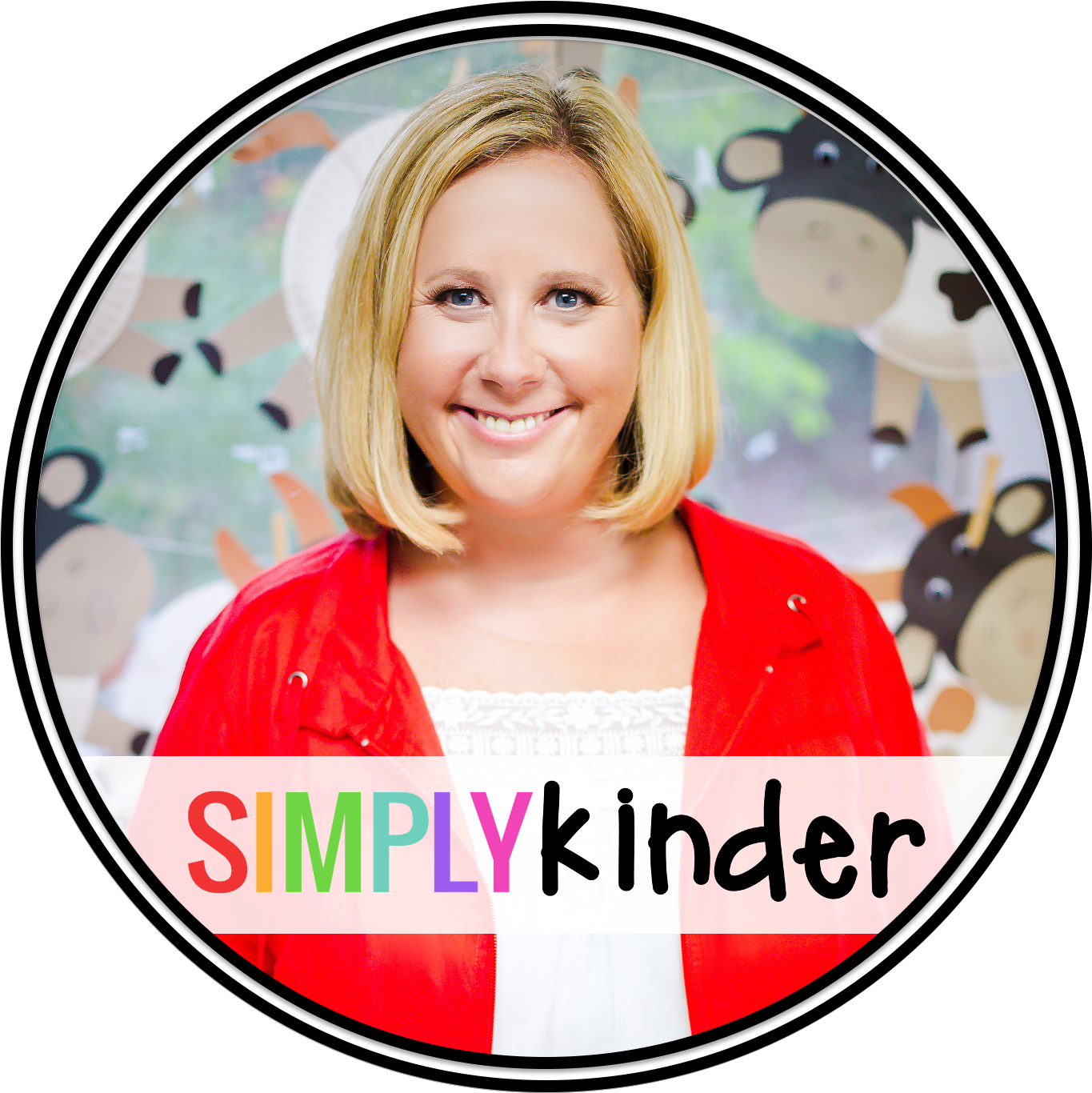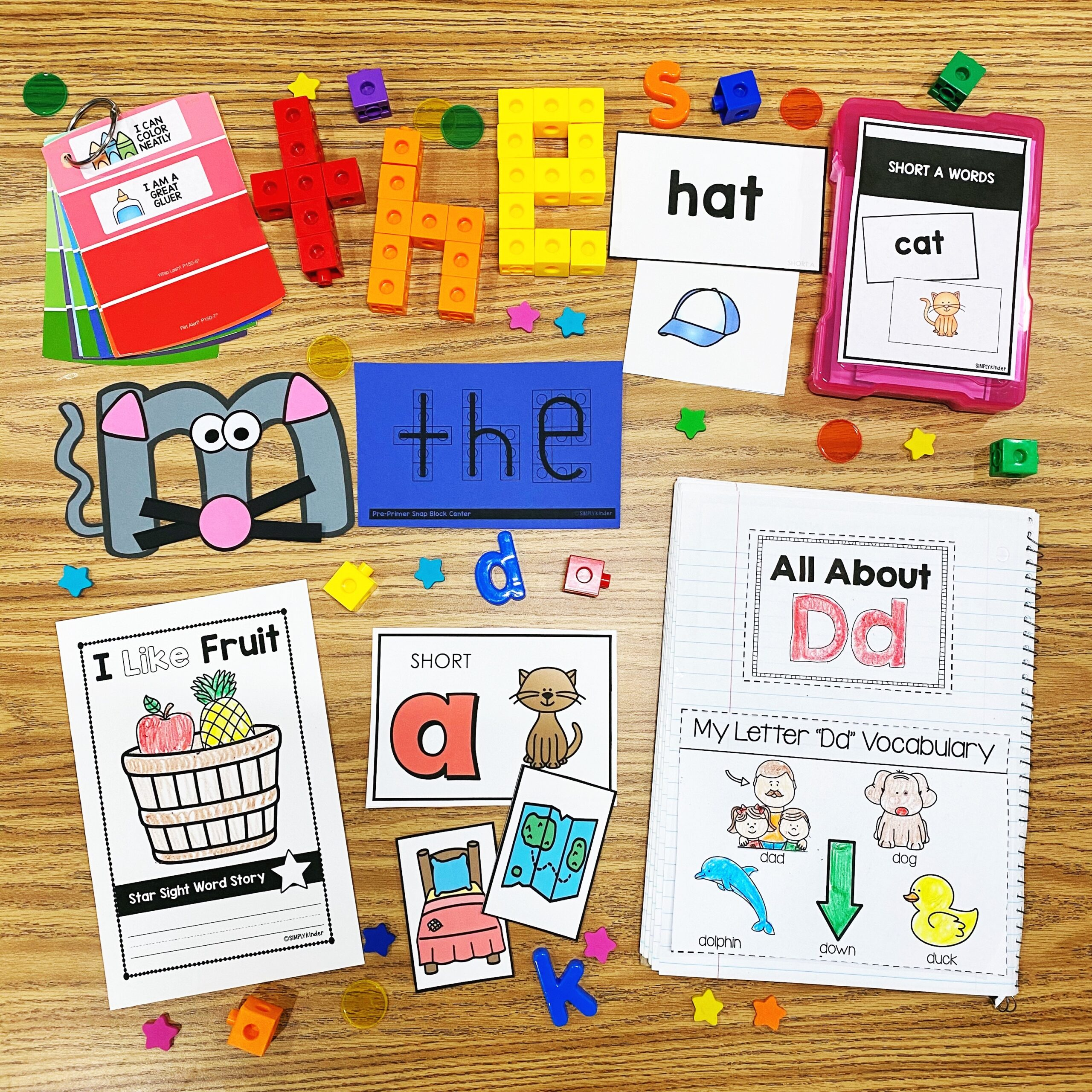Math Word Problems That Will Delight Your Kinders - Simply Kinder

# Math Word Problems That Will Delight Your Kinders

Looking for some fun and engaging FREE math word problems for Kindergarten students? Children will practice addition and subtraction with our fun monster math word problems. Snag your free printable pack today.

## This Activity Pairs Well With

It can be a lot of fun teaching math but teaching word problems can be particularly challenging. There are all those words for a start!

Then students also need to draw upon a multitude of skills to succeed. They need to read and understand the text. Pull out the important information and then do the math correctly.

That is quite the task for young learners who are just developing there reading and math skills. Still, there is value in starting to do this at the Kindergarten level. Sure, children could just memorise math facts, but this will only take them so far. What we want our kids to be able to tackle any math problem with confidence, because they had a good understanding of how our number system works.

## The Benefits of doing Math Word Problems

Providing opportunities for students to engage in problem solving has multiple benefits. It helps

• Develop mathematical reasoning.
• Provides opportunities to communicate mathematical thinking.
• Gives ongoing practice with specific operations.
• Keeps math interesting and relevant.

Before we begin, let’s look at the basics.

## What Is a Math Word Problem?

A math word problem consists of a few sentences describing a scenario that needs to be solved using a mathematical calculation. This means that the important information is presented as text rather than just numbers.

As Kinders are concrete thinkers we start by asking students to use manipulatives to act out the scenarios. This allows them to see so they can see and experience the word problems.

## What is the Best Way for Kinders to Solve Math Word Problems?

There are five main steps

• Circle or underline all the numbers and underline the important words.
• Use manipulatives or draw the problem. The manipulatives can be as simple as paper images.
• Write the number sentence.
• Solve the number problem.

## Free Printable Worksheets

We’ve created a pack of free printable worksheets to help students practice math word problems. There are four worksheets to cover different types of word problem.

The worksheets use several different tools to help students solve the problem. They include paper manipulatives that must be cut out. One of the worksheets uses a ten frames and others have number lines. Every worksheet encourages the students to write down the number sentence after they have acted it out with the manipulatives.

The manipulatives allow the students to see the problem unfold. Focus on the vocabulary as you start to do word problems. Students need to learn which words tell them how to do the math.

An added bonus of using worksheets like these is that you can see how your students solved the problem. It is easy to see if they make a mistake and to go from there.

Don’t forget there is often more than one way to solve a math problem. Celebrate students that come up with new ways to do the math. Invite them to share their method with the class.

## A Closer Look At The Worksheets

Our worksheets feature some cute monsters. Most children love monsters, and these ones are particularly appealing. In each case the students will cut out the manipulatives at the bottom of the page and then use them to work through the problem.

The manipulatives are glued into place. Then children are asked to turn the word problem into an equation and provide an answer. Of course, everything can be colored at the end.

We have 5 worksheets. Four of them feature different kids of word problems and the last one is a blank for children to create their own word problem.

#### Worksheet 1 a “Put Together” Problem (Addition)

These are the simplest math word problems and are easily solved with paper manipulatives. Children will cut out the required number of candies, glue them to the picture and count the total. They can also use the number line.

Next, we have an add on problem featuring a monster and her pet spiders. Children can use the number line to count on. They will also cut out the spiders and count them.

#### Worksheet 3 a “Take From” Problem (Subtraction)

The third worksheet starts with the total number and takes some away. Students will cut out 8 candies, place them down and then remove 3 from the jar. The remaining candies can be glued down.

#### Worksheet 4 a “Take Apart” Problem

These problems start with the total. We then break up the total into two or more smaller groups.

These are the hardest kinds of word problems for Kinders to tackle. The worksheet provides a space to act out the problem. Children can cut out 4 hearts, color 6 of them red and the rest pink. We’ve also included a ten square. Use buttons or the paper hearts. Then write the equation and solve it.

The great thing about these story problems is that students are practicing their math skills, but they are also working on reading and making sure they are understanding the question (comprehension).

## How can I Use These Worksheets?

They would be great for morning work or homework. You could also use them as math warmups. Pair kids up to work on the problems together or send them home as homework.

## Common Core Connections

If you follow the common core, these worksheets cover the following standards.

1. Represent addition and subtraction with objects, fingers, mental images, drawings1, sounds (e.g., claps), acting out situations, verbal explanations, expressions, or equations.
2. Solve addition and subtraction word problems, and add and subtract within 10, e.g., by using objects or drawings to represent the problem.
3. Decompose numbers less than or equal to 10 into pairs in more than one way, e.g., by using objects or drawings, and record each decomposition by a drawing or equation.

## Need More Math Activities?Welcome! It is our goal to help busy teachers just like you to be the best teachers they can be through our classroom ideas, resources, and community. ~ Jennifer

## Join the ListJoin thousands of other teachers and stay on top of all things kindergarten.  Receive a FREE 46 page resource to get started!

## NEW RESOURCES